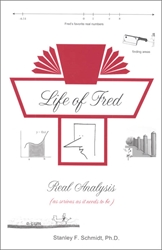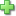# Life of Fred: Real Analysis

by Stanley F. Schmidt
Price: \$49.00

Real Analysis is a topic studied by math majors in college. The math contained herein is more advanced than what most non-majors ever see, but it still has Fred, and he has a very good time with his favorite topic. This program is college level, meant to be used only after algebra, geometry, and calculus courses are mastered. Real Analysis takes real numbers that were studied in algebra, and functions and limits that were studied in calculus, and asks the student to analyze them using the process of proofs that were studied in geometry. These proofs Dr. Schmidt calls "puzzles", and at this level, the idea is not so much to solve problems but to understand concepts. As a result, some of them will be short and straightforward, while others will take some time. Answers to all 113 puzzles are included in the back of the book.

Calculus is a requirement before studying this topic, and Life of Fred: Five Days is recommended.

Topics include:

• The Real Numbers
• Sequences
• Series
• Tests for Series Convergence
• Limits and Continuity
• Derivatives
• The Riemann Integral
• Sequences of Functions
• Series of Functions
• Two Cow-Moving Theorems
• Looking Ahead to Topics beyond a First Course in Real Analysis.

The Real Numbers, Sequences, Series, Tests for Series Convergence, Limits and Continuity, Derivatives, the Riemann Integral, Sequences of Functions, Series of Functions, and Looking Ahead to Topics beyond a First Course in Real Analysis.

Subtopics include: The axiomatic approach to the real numbers, eleven properties of the real numbers, mathematics after calculus, definition of a function, if a and b are irrational, must ab also be irrational?, two definitions of dense subsets, the natural numbers are well-ordered, the positive real numbers are Archimedean—two definitions, math induction proofs, one-to-one (injective) functions, cardinality of a set, four definitions of onto, finding a one-to-one onto function from (0, 1) to [0, 1], countable and uncountable sets, Root Test, Ratio Test, Integral Test, absolute and conditional convergence, weak and strong induction proofs, secant lines, limit proofs using ε and δ, eight theorems about limits and their proofs, lim g(f(x)) does not always equal g(lim f(x)), continuous functions, four theorems about pairs of continuous functions, the squeeze theorem, a very short proof that lim sin x = 0 as x approaches zero, two definitions of derivative, the delta process, the five standard derivative rules and their proofs, how much detail to put in a proof, Schwarzschild radii, converses, contrapositives, and inverses, Intermediate Value Theorem, Rolle’s theorem, Mean Value Theorem, L’Hospital’s rule, proving lim (sin θ)/θ = 1 in two steps, detailed definition of the Riemann integral, uniform continuity, Fundamental Theorem of Calculus, Cauchy sequence of functions, Cauchy series of functions, uniform convergence of a series of functions, Weierstrass M-test, power series, two formulas for the radius of convergence, taking derivatives and antiderivatives of a power series, Weierstrass Approximation theorem, finding an approximation for ln 5 on a desert island, and the Cantor set.

Did you find this review helpful?
Related Categories
Recommended for...
Customer ReviewsClick here to write a review